# NCERT Solutions for Class 6 Maths Chapter 8 – Decimals

NCERT Solutions for Class 6 Maths Chapter 8 – Decimals comprises of the 6 Exercises

This Chapter contains the Exercises relating to the following topics, which are discussed in Chapter 8 – Decimals Class 6 NCERT book  : –

• 8.1 Introduction
• 8.2 Tenths
• 8.3 Hundredths
• 8.4 Comparing Decimals
• 8.5 Using Decimals
• 8.5.1 Money
• 8.5.2 Length
• 8.5.3 Weight
• 8.6 Addition of Numbers with Decimals
• 8.7 Subtraction of Decimals

### NCERT Solutions for Class 6 Maths Chapter 8 Exercise 8.1

Ques. 1. Write the following as numbers in the given table.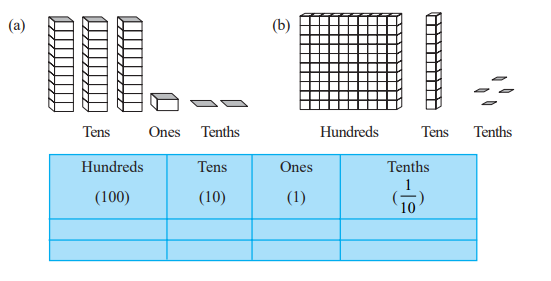Sol. 1.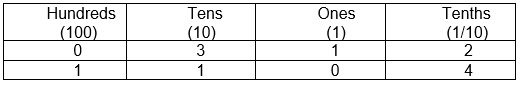Ques. 2. Write the following decimals in the place value table.
(a) 19.4
(b) 0.3
(c) 10.6
(d) 205.9

Sol. 2.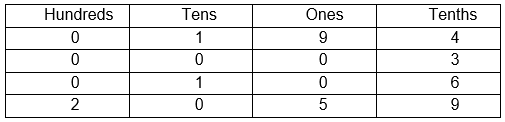Ques. 3. Write each of the following as decimals :

(a) Seven-tenths
(b) Two tens and nine-tenths
(c) Fourteen point six
(d) One hundred and two ones
(e) Six hundred point eight

Sol. 3. (a) 7 × 1/10 = 0.7
(b) 2 × 10 + 9 × 1/10 = 20.9
(c) 14.6
(d) 100 + 2 × 1 =102
(e) 600.8

Ques. 4. Write each of the following as decimals:
(a) 5/10
(b) 3 + 7/10
(c) 200 + 60 + 5 + 1/10
(d) 70 + 8/10
(e) 88/10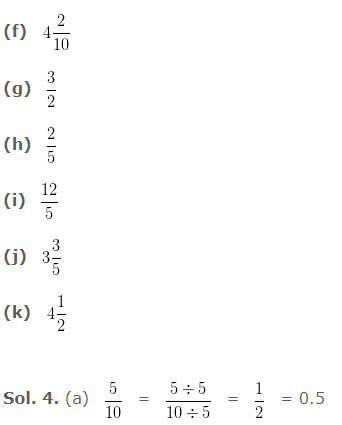(b) 3 + 7/10 = 3 + 0.7 = 3.7
(c) 200 + 60 + 5 + 1/10 = 265 + 0.1 = 265.1
(d) 70 + 8/10 = 70 + 0.8 = 70.8
(e) 88/10 = 8.8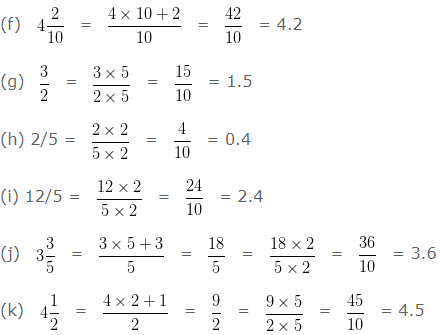Ques. 5. Write the following decimals as fractions. Reduce the fractions to lowest form.
(a) 0.6
(b) 2.5
(c) 1.0
(d) 3.8
(e) 13.7
(f) 21.2
(g) 6.4

Sol. 5. (a) 0.6
= 6/10
= 3/5

(b) 2.5
= 25/10
= 5/2

(c) 1.0
= 10/10
= 1

(d) 3.8
=38/10
= 19/5

(e) 13.7
= 137/10

(f) 21.2
= 212/10
= 106/5

(g) 6.4
= 64/10
= 32/5

Ques. 6. Express the following as cm using decimals.
(a) 2 mm
(b) 30 mm
(c) 116 mm
(d) 4 cm 2 mm
(e) 162 mm
(f) 83 mm

Sol. 6. We know that, 1 cm = 10 mm

(a) 2 mm
= 2/10  cm
= 0.2 cm

(b) 30 mm
= 30/10
= 3 cm

(c) 116 mm
= 116/10 cm
= 11.6 cm

(d) 4 cm 2 mm
= 4 cm + 2/10 cm
= 4.2 cm

(e) 162 mm
= 162/10 cm
= 16.2 cm

(f) 83 mm
= 83/10 cm
= 8.3 cm

Ques. 7. Between which two whole numbers on the number line are the given numbers lie? Which of these whole numbers is nearer the number?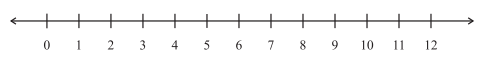(a) 0.8
(b) 5.1
(c) 2.6
(d) 6.4
(e) 9.1
(f) 4.9

Sol. 7. (a) 0.8 – It lies between 0 to 1 and the nearer number is 1.
(b) 5.1 – It lies between 5 to 6 and the nearer number is 5.
(c) 2.6 – It lies between 2 to 3 and the nearer number is 3.
(d) 6.4 – It lies between 6 to 7 and the nearer number is 6.
(e) 9.1 – It lies between 9 to 10 and the nearer number is 9.
(f) 4.9 – It lies between 4 to 5 and the nearer number is 5.

Ques. 8. Show the following numbers on the number line.
(a) 0.2
(b) 1.9
(c) 1.1
(d) 2.5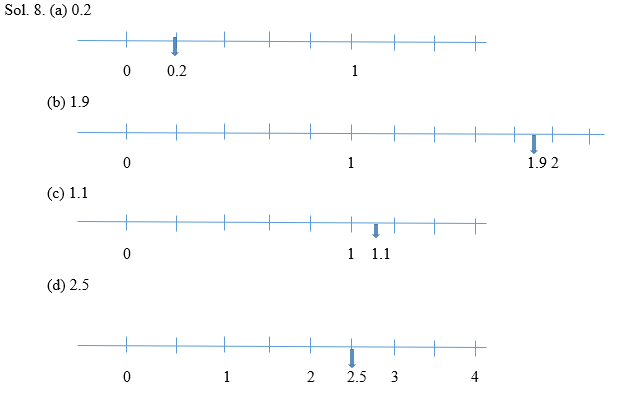Ques.  9. Write the decimal number represented by the points A, B, C, D on the given number line.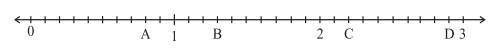Sol. 9. (a) A = 0.8
(b) B = 1.3
(c) C = 2.2
(d) D = 2.9

Ques. 10. (a) The length of Ramesh’s notebook is 9 cm 5 mm. What will be its length in cm?
(b) The length of a young gram plant is 65 mm. Express its length in cm.

Sol. 10. (a) Length of Ramesh’s notebook =  9 cm 5 mm
Length in cm  = 9 + [5/10] = 9.5 cm

(b) length of a young gram plant   =   65 mm
Length in cm =   65/10 = 6.5 cm

### NCERT Solutions for Class 6 Maths Chapter 8 Exercise 8.2

Ques. 1. Complete the table with the help of these boxes and use decimals to write the number.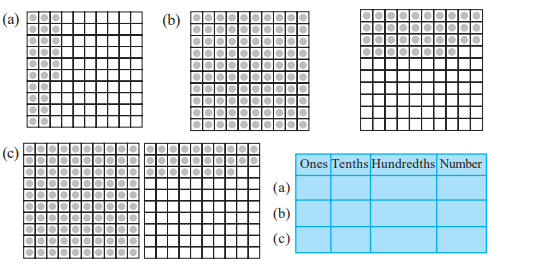Sol. 1.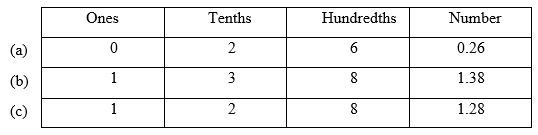Ques. 2. Write the numbers given in the following place value table in decimal form.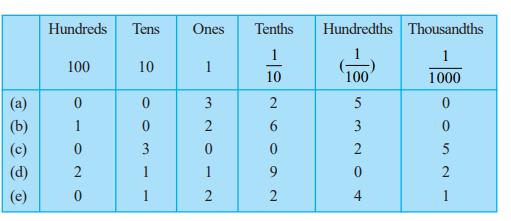Sol. 2. (a) 3 + 2/10 + 5/100 = 3 + 0.2 + 0.05 = 3.25
(b) 100 + 2 + 6/10 + 3/100 = 100 + 2 + 0.6 + 0.03 = 102.63
(c) 30 + 2/100 + 5/1000 = 30 + 0.02 + 0.005 = 30.025
(d) 200 + 10 + 1 + 9/10 + 2/1000 = 200 + 10 + 1 + 0.9 + 0.002 = 211.902
(e) 10 + 2 + 2/10 + 4/100 + 1/1000 = 10 + 2 + 0.2 + 0.04 + 0.001 = 12.241

Ques. 3. Write the following decimals in the place value table.
(a) 0.29
(b) 2.08
(c) 19.60
(d) 148.32
(e) 200.812

Sol. 3.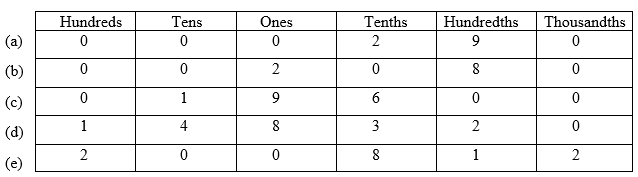The above table has been formed using the following method:
(a) 0.29 = 0 + 2/10 + 9/100
(b) 2.08 = 2 + 0/10 + 8/100
(c) 19.60 = 10 + 9 + 6/10 + 0/100
(d) 148.32 = 100 + 40 + 8 + 3/10 + 2/100
(e) 200.812 = 200 + 8/10 + 1/100 + 2/1000

Ques. 4. Write each of the following as decimals.
(a) 20 + 9 + 4/10 + 1/100
(b) 137 + 5/100
(c) 7/10 + 6/100 + 4/1000
(d) 23 + 2/10 + 6/1000
(e) 700 + 20 + 5 + 9/100

Sol. 4. (a) 20 + 9 + 4/10 + 1/100 = 20 + 9 + 0.4 + 0.01 = 29.41
(b) 137 + 5/100 = 137 + 0.05 = 137.05
(c) 7/10 + 6/100 + 4/1000 = 0.7 + 0.06 + 0.004 = 0.764
(d) 23 + 2/10 + 6/1000 = 23 + 0.2 + 0.006 = 23.206
(e) 700 + 20 + 5 + 9/100 = 700 + 20 + 5 + 0.09 = 725.09

Ques. 5. Write each of the following decimals in words.
(a) 0.03
(b) 1.20
(c) 108.56
(d) 10.07
(e) 0.032
(f) 5.008

Sol.5.   (a) 0.03 Zero point zero three
(b) 1.20 One point two zero
(c) 108.56 One hundred eight point five six
(d) 10.07 Ten point zero seven
(e) 0.032 Zero point zero three two
(f) 5.008 Five point zero zero eight

Ques. 6. Between which two numbers in tenths place on the number line does each of the given number lie?
(a) 0.06
(b) 0.45
(c) 0.19
(d) 0.66
(e) 0.92
(f) 0.57

Sol.6.   (a) 0.06 It lies between 0 and 0.1
(b) 0.45 It lies between 0.4 and 0.5
(c) 0.19 It lies between 0.1 and 0.2
(d) 0.66 It lies between 0.6 and 0.7
(e) 0.92 It lies between 0.9 and 1.0
(f) 0.57 It lies between 0.5 and 0.6

Ques. 7. Write as fractions in lowest terms.
(a) 0.60
(b) 0.05
(c) 0.75
(d) 0.18
(e) 0.25
(f) 0.125
(g) 0.066

Sol.7.   (a) 0.60 = 60/100
= 6/10
= 3/5

(b) 0.05 = 5/100
= 1/20

(c) 0.75 = 75/100
= 3/4

(d) 0.18 = 18/100
= 9/50

(e) 0.25 = 25/100
= 1/4

(f) 0.125 = 125/1000
= 5/40
= 1/8

(g) 0.066 = 66/1000
= 33/500

### NCERT Solutions for Class 6 Maths Chapter 8 Exercise 8.3

Ques. 1. Which is greater?
(a) 0.3 or 0.4
(b) 0.07 or 0.02
(c) 3 or 0.8
(d) 0.5 or 0.05
(e) 1.23 or 1.2
(f) 0.099 or 0.19
(g) 1.5 or 1.50
(h) 1.431 or 1.490
(i) 3.3 or 3.300
(j) 5.64 or 5.603

Sol. 1. (a) 0.3 and 0.4
Before the decimal point, both the digits are same.
After the decimal point, the digit with larger value, gives a greater number.
Hence, 0.4 > 0.3.

(b) 0.07 and 0.02
Before the decimal point, both the digits are same.
After the decimal point, the digit with larger value, gives a greater number.
Hence, 0.07 > 0.02

(c) 3 and 0.8
Before the decimal point, the digit with greater value, gives a greater number.
Hence, 3 > 0.8

(d) 0.5 and 0.05
The closest digit after the decimal point is compared with that of the other number. The one with larger value, gives a greater number.
Hence, 0.5 > 0.05

(e) 1.23 and 1.2
Before the decimal point, both the digits are same.
After the decimal point, the digit with larger value, gives a greater number.
Here, the closest digit after the decimal point is same in both the numbers.
So, the next digit is compared and the one with greater value is a greater number.
Hence, 1.23 > 1.2

(f) 0.099 and 0.19
Before the decimal point, both the digits are same.
The closest digit after the decimal point is compared with that of the other number. The one with larger value, gives a greater number.
Hence, 0.19 > 0.099

(g) 1.5 and 1.50
When a digit 0 is at the last place in a decimal number then, its value is considered to be nothing.
Hence, 1.5 = 1.50

(h) 1.431 and 1.490
Before the decimal point, both the digits are same.
After the decimal point, the digit with larger value, gives a greater number.
Here, the closest digit after the decimal point is same in both the numbers.
So, the next digit is compared and the one with greater value gives a greater number.
Hence, 1.490 > 1.431

(i) 1.3 and 1.300
When a digit 0 is at the last place in a decimal number then, its value is
considered to be nothing.
Hence 1.3 = 1.300

(j) 5.64 or 5.603
Before the decimal point, both the digits are same.
After the decimal point, the digit with larger value, gives a greater number.
Here, the closest digit after the decimal point is same in both the numbers.
So, the next digit is compared and the one with greater value gives a greater number.
Hence 5.64 > 5.603

Ques. 2. Make five more examples and find the greater number from them.

Sol. 2. (a) 1.25 and 1.63
Before the decimal point, both the digits are same.
The closest digit after the decimal point is compared with that of the other number. The one with larger value, gives a greater number.
Hence, 1.63 > 1.25

(b) 3.02 and 4.07
Before the decimal point, the digit with greater value, gives a greater number.
Hence, 4.07 > 3.02

(c) 1.60 and 1.600
When a number 0 is at the last place in a decimal number then, its value is considered to be nothing.
Hence, 1.60 = 1.600

(d) 2.02 and 2.2
Before the decimal point, both the digits are same.
The closest digit after the decimal point is compared with that of the other number. The one with larger value, gives a greater number.
Hence, 2.2 > 2.02

(e) 25.63 and 0.63
Before the decimal point, the digit with greater value, gives a greater number.
Hence, 25.63 > 0.63

### NCERT Solutions for Class 6 Maths Chapter 8 Exercise 8.4

Ques. 1. Express as rupees using decimals.
(a) 5 paise
(b) 75 paise
(c) 20 paise
(d) 50 rupees 90 paise
(e) 725 paise

Sol. 1.  (a) 5 paise
1 rupee = 100 paise
1 paise = 1/100 rupee
Hence, 5 paise = 5/100 rupee = 0.05 rupees

(b) 75 paise
1 rupee = 100 paise
1 paise = 1/100 rupee
Hence, 75 paise = 75/100 rupee = 0.75 rupees

(c) 20 paise
1 rupee = 100 paise
1 paise = 1/100 rupee
Hence, 20 paise = 20/100 rupee = 0.20 rupees.

(d) 50 rupees 90 paise
1 rupee = 100 paise
1 paise = 1/100 rupee
Hence, 50 rupee 90 paise = 50 + 90/100 rupee = 50 + 0.90 rupees = 50.90 rupees.

(e) 725 paise
1 rupee = 100 paise
1 paise = 1/100 rupee
Hence, 725 paise = 725/100 rupees = 7.25 rupees.

Ques. 2. Express as metres using decimals.

(a) 15 cm
(b) 6 cm
(c) 2 m 45 cm
(d) 9 m 7 cm
(e) 419 cm

Sol. 2. (a) 15cm
1 m = 100cm
1 cm = 1/100 m
Hence, 15 cm = 15/100 m = 0.15 m

(b) 6cm
1 m = 100cm
1 cm = 1/100 m
Hence, 6 cm = 6/100 m = 0.06 m

(c) 2m 45cm
1 m = 100 cm
1 cm = 1/100 m
Hence, 2 m 45 cm = 2 + 45/100 m = 2 + 0.45 m = 2.45 m

(d) 9m 7cm
1 m = 100cm
1 cm = 1/100 m
Hence, 9 m 7 cm = 9 + 7/100 m = 9 + 0.07 m = 9.07 m

(e) 419cm
1 m = 100cm
1 cm = 1/100 m
Hence, 419 cm = 419/100 m = 4.19 m

Ques. 3. Express as cm using decimals.
(a) 5 mm
(b) 60 mm
(c) 164 mm
(d) 9 cm 8 mm
(e) 93 mm

Sol. 3. (a) 5mm
1cm = 10mm
1 mm = 1/10 cm
Hence, 5 mm = 5/10 cm = 0.5 cm

(b) 60mm
1cm = 10mm
1 mm = 1/10 cm
Hence, 60 mm = 60/10 cm = 6 cm

(c) 164mm
1cm = 10mm
1 mm = 1/10 cm
Hence, 164 mm = 164/10 cm = 16.4 cm

(d) 9cm 8mm
1cm = 10mm
1 mm = 1/10 cm
Hence, 9cm 8mm = 9 + 8/10 cm = 9 + 0.8 cm = 9.8 cm

(e) 93mm
1cm = 10mm
1 mm = 1/10 cm
Hence, 93 mm = 93/10 cm = 9.3 cm

Ques. 4. Express as km using decimals.
(a) 8 m
(b) 88 m
(c) 8888 m
(d) 70 km 5m

Sol. 4. (a) 8m
1km = 1000m
1 m = 1/1000 km
Hence, 8 m = 8/1000 km = 0.008 km

(b) 88m
1km = 1000m
1 m = 1/1000 km
Hence, 88 m = 88/1000 km = 0.088 km

(c) 8888
1km = 1000m
1 m = 1/1000 km
Hence, 8888 m = 8888/1000 km = 8.888 km

(d)70km 5m
1km = 1000m
1 m = 1/1000 km
Hence, 70 km 5 m = 70 + 5/1000 km = 70 + 0.005 km = 70.005 km

Ques. 5. Express as kg using decimals.
(a) 2 g
(b) 100 g
(c) 3750 g
(d) 5 kg 8 g
(e) 26 kg 50 g

Sol. 5. (a) 2g
1kg = 1000g
1 g = 1/1000 kg
Hence, 2g = 2/1000 kg = 0.002 kg

(b) 100g
1kg = 1000g
1 g = 1/1000 kg
Hence, 100g = 100/1000 kg = 0.100 kg

(c) 3750g
1kg = 1000g
1 g = 1/1000 kg
Hence, 3750g = 3750/1000 kg = 3.750 kg

(d) 5kg 8g
1kg = 1000g
1 g = 1/1000 kg
Hence, 5kg 8g = 5 + 8/1000 kg = 5 + 0.008 kg = 5.008kg

(e) 26kg 50g
1kg = 1000g
1 g = 1/1000 kg
Hence, 26kg 50g = 26 + 50/1000 kg = 26 + 0.050 kg = 26.050 kg

### NCERT Solutions for Class 6 Maths Chapter 8 Exercise 8.5

Ques. 1. Find the sum in each of the following:
(a) 0.007 + 8.5 + 30.08
(b) 15 + 0.632 + 13.8
(c) 27.076 + 0.55 + 0.004
(d) 25.65 + 9.005 + 3.7
(e) 0.75 + 10.425 + 2
(f) 280.69 + 25.2 + 38

Sol. 1. (a) 0.007 + 8.5 + 30.08 = 0.007 + 8.500 + 30.080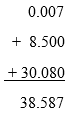(b) 15 + 0.632 + 13.8 = 15.000 + 0.632 + 13.800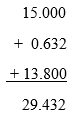(c) 27.076 + 0.55 + 0.004 = 27.076 + 0.550 + 0.004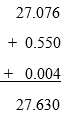(d) 25.65 + 9.005 + 3.7 = 25.650 + 9.005 + 3.700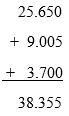(e) 0.75 + 10.425 + 2 = 0.750 + 10.425 + 2.000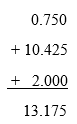(f) 280.69 + 25.2 + 38 = 280.690 + 25.200 + 38.000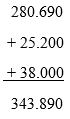Ques. 2. Rashid spent Rs 35.75 for Maths book and Rs 32.60 for Science book. Find the total amount spent by Rashid

Sol. 2. Amount spent by Rashid for Maths book  =    Rs 35.75
Amount spent by Rashid for Science book  =    Rs 32.60
Total amount spent by Rashid =  Rs. 35.75 + Rs. 32.60 =  Rs 68.35

Ques. 3. Radhika’s mother gave her Rs 10.50 and her father gave her Rs 15.80, find the total amount given to Radhika by the parents.

Sol. 3. Amount Radhika’s mother given her  =  Rs 10.50
Amount Radhika’s father given her  =   Rs 15.80
Total amount given to Radhika by the parents =  Rs. 10.50 + Rs. 15.80 =  Rs 26.30

Ques. 4. Nasreen bought 3 m 20 cm cloth for her shirt and 2 m 5 cm cloth for her trouser. Find the total length of cloth bought by her.

Sol. 4. Length of cloths Nasreen bought for her shirt  = 3m 20cm
Length of cloths Nasreen bought for her trouser =  2m 5cm
total length of cloth bought by her = 3m 20cm + 2m 5cm =    5m 25 cm

Ques. 5. Naresh walked 2 km 35 m in the morning and 1 km 7 m in the evening. How much distance did he walk in all?

Sol. 5. Distance Naresh walked in morning =  2km 35m
Distance he walked in evening  =    1km 7m
Total distance he walk in all =  2km 35m + 1km 7m =  3km 42m

Ques. 6. Sunita travelled 15 km 268 m by bus, 7 km 7 m by car and 500 m on foot in order to reach her school. How far is her school from her residence?

Sol. 6. Distance Sunita travelled by bus = 15km 268m
Distance Sunita travelled by car   =  7km   7m
Distance Sunita travelled on foot  =  0km 500m
Distance from her school to her residence  =  15km 268m + 7km 7m + 0km 500 m = 22km 775m

Ques. 7. Ravi purchased 5 kg 400 g rice, 2 kg 20 g sugar and 10 kg 850g flour. Find the total weight of his purchases.

Sol. 7. Weight of rice Ravi purchased  =  5kg 400g
Weight of sugar he purchased  =  2kg  20g
Weight of flour he purchased   =  10kg 850g
Total weight of his purchases   =  5kg 400g + 2kg  20g + 10kg 850g = 18kg 270g

### NCERT Solutions for Class 6 Maths Chapter 8 Exercise 8.6

Ques.1. Subtract :

(a) Rs 18.25 from Rs 20.75
(b) 202.54 m from 250 m
(c) Rs 5.36 from Rs 8.40
(d) 2.051 km from 5.206 km
(e) 0.314 kg from 2.107 kg

Sol. 1. (a) Rs 18.25 from Rs 20.75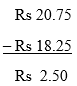(b) 202.54m from 250.00m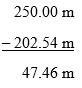(c) Rs 5.36 from 8.40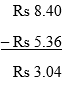(d) 2.051km from 5.206km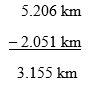(e) 0.314 kg from 2.107 kg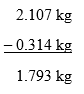Ques. 2. Find the value of :
(a) 9.756 – 6.28
(b) 21.05 – 15.27
(c) 18.5 – 6.79
(d) 11.6 – 9.847

Sol. 2.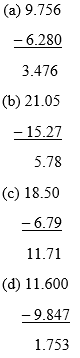Ques. 3. Raju bought a book for Rs 35.65. He gave Rs 50 to the shopkeeper. How much money did he get back from the shopkeeper?

Sol. 3. Amount Raju gave to the shopkeeper  =  Rs 50.00
Amount for the book Raju bought  =  Rs 35.65
Amount he will get back from the shopkeeper =  Rs 50.00 – Rs 35.65 = Rs 14.35

Ques. 4. Rani had Rs 18.50. She bought one ice-cream for Rs 11.75. How much money does she have now?

Sol. 4. Money Rani had = Rs 18.50
Money she spent on ice-cream = Rs  11.75
Money she has now = Rs 18.50 – Rs  11.75 = Rs   6.75

Ques. 5. Tina had 20 m 5 cm long cloth. She cuts 4 m 50 cm length of cloth from this for making a curtain. How much cloth is left with her?

Sol. 5. Length of cloth Tina had  =  20m 05cm
Length of cloth she cut for making curtain =  4m 50cm
Length of cloth left with her  =  20m 05cm –  4m 50cm = 15m 55cm

Ques. 6. Namita travels 20 km 50 m every day. Out of this she travels 10 km 200 m by bus and the rest by auto. How much distance does she travel by auto?

Sol. 6. Distance Namita travels every day = 20km 050m
Distance she travels by bus = 10km 200m
Distance she travels by auto = 20km 050m – 10km 200m = 9km 850m

Ques. 7. Aakash bought vegetables weighing 10 kg. Out of this, 3 kg 500 g is onions, 2 kg 75g is tomatoes and the rest is potatoes. What is the weight of the potatoes?

Sol. 7. Weight of onions Aakash bought = 3kg 500g
Weight of tomatoes he bought  = 2kg 075g
Total weight of onions and tomatoes he bought  =  3kg 500g + 2kg 075g = 5kg 575g
Total weight of vegetable Aakash bought  =  10kg 000g
Total weight of onions and tomatoes he bought  = 05kg 575g
Weight of potatoes he bought  =  10kg 000g –  05kg 575g = 4kg 425g

With this we come to the end of NCERT Solutions for Class 6 Maths Chapter 8 Decimals. We hope these helped you study your subject.